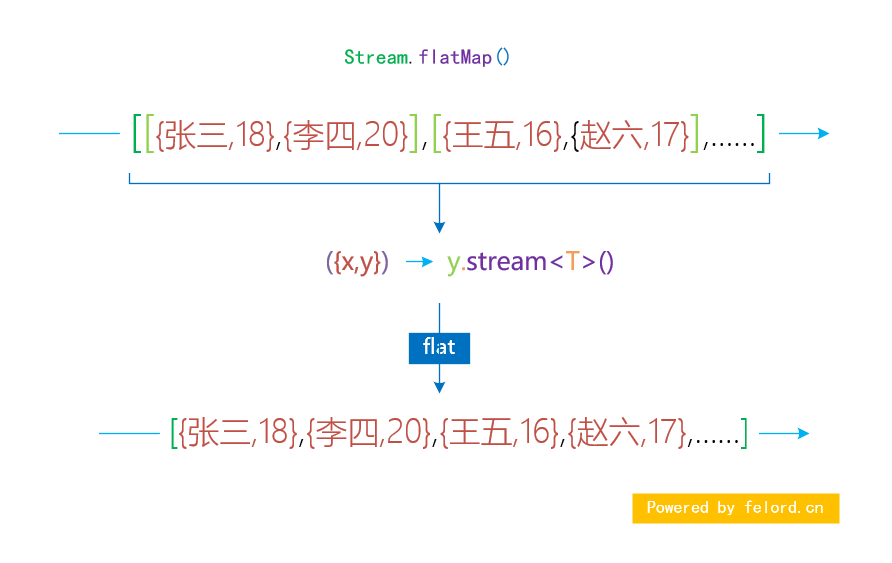# Java Stream 流的合并操作

## 1. 前言

Java Stream Api 提供了很多有用的 Api 让我们很方便将集合或者多个同类型的元素转换为流进行操作。今天我们来看看如何合并 Stream 流。

## 2. Stream 流的合并

Stream 流合并的前提是元素的类型能够一致。

### 2.1 concat

``````Stream<Integer> stream = Stream.of(1, 2, 3);
Stream<Integer> another = Stream.of(4, 5, 6);
Stream<Integer> concat = Stream.concat(stream, another);

List<Integer> collect = concat.collect(Collectors.toList());
List<Integer> expected = Lists.list(1, 2, 3, 4, 5, 6);

Assertions.assertIterableEquals(expected, collect);``````### 2.2 多个流的合并

``Stream.concat(Stream.concat(stream, another), more);````````Stream<Integer> stream = Stream.of(1, 2, 3);
Stream<Integer> another = Stream.of(4, 5, 6);
Stream<Integer> third = Stream.of(7, 8, 9);
Stream<Integer> more = Stream.of(0);
Stream<Integer> concat = Stream.of(stream,another,third,more).
flatMap(integerStream -> integerStream);
List<Integer> collect = concat.collect(Collectors.toList());
List<Integer> expected = Lists.list(1, 2, 3, 4, 5, 6, 7, 8, 9, 0);
Assertions.assertIterableEquals(expected, collect);``````

### 2.3 第三方库

``````List<Integer> block = Flux.fromStream(stream)
.mergeWith(Flux.fromStream(another))
.collectList()
.block();``````